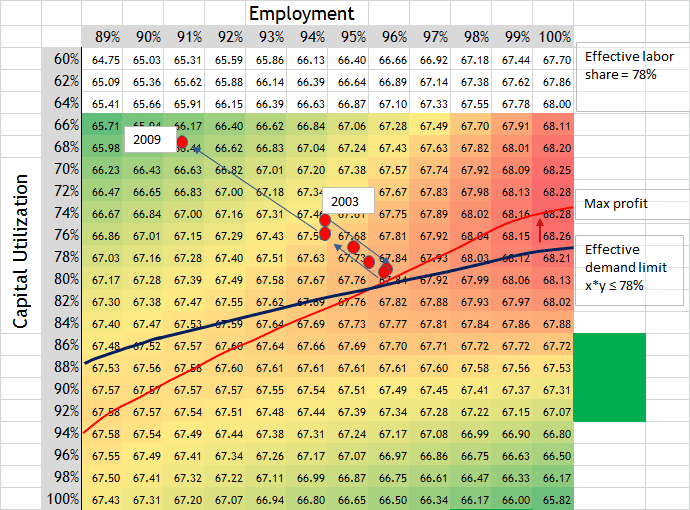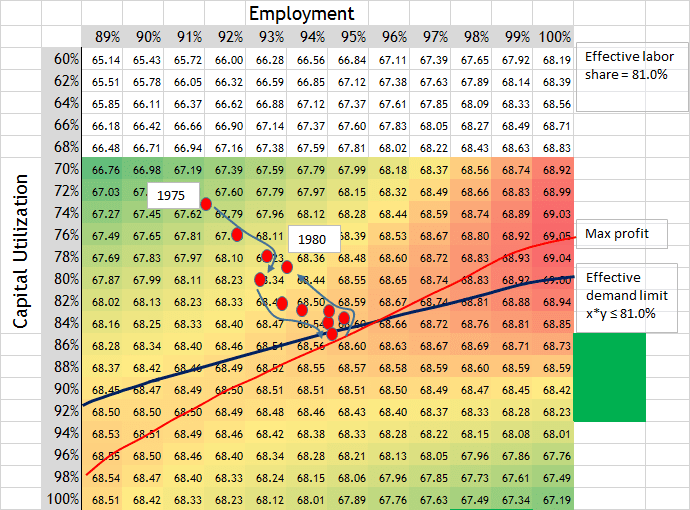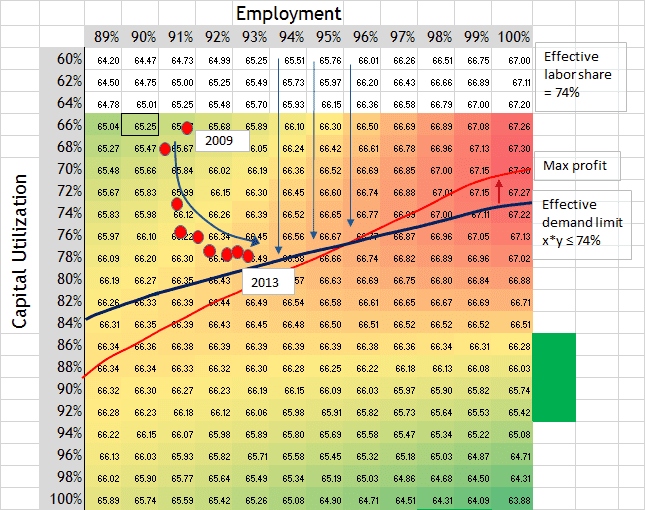## The Cobra Equation for the Effective Demand business cycle (part 2)

OK… so the exploration is continuing into the new equation which I am calling “The Cobra Equation” because the 3D curve looks like a cobra. This equation shows promise in its ability to explain the process of the business cycle and to ascertain the utilization of labor and capital near the end of the business cycle.I have simplified the equation to this form…

Measure of profitability = MFP * ((x + y) – a * (x^2 * y^2))

MFP = marginal factor productivity (assumed \$50 in video).
x = capital utilization rate
y = employment rate
a = coefficient of labor share to establish effective demand limit on capacity utilization. Coefficient a = 0.88*s^2 – 2.31*s + 1.95 … (s = effective labor share, for example 80% as 0.80).

I plotted the equation for the current effective labor share of 74%. And made a video. (source of 3D graphic in video.)

Notes to video: The constraint is x*y ≤ effective labor share. I say 74% in the video, but effective labor share can change. Also, the numbers in the Excel spreadsheet include the MFP, marginal factor productivity of \$50, in the equation. The 3D graphic equation did not include the MFP.

The video explains how the economy is moving within the constraint of the effective demand limit as firms reach for profits along the profit maximization line. The capacity utilization rate will stay steady or decrease as the economy moves forward toward the end of a business cycle. For example, capacity utilization has already stayed constant for almost 2 years. We may be sliding backwards as far as capacity utilization. This equation is new so we have to watch it.

Even so, the video explains the process of the business cycle moving in a circular motion on the plot.

Other examples…

Here is a graph for the data before the 2008 crisis, when the effective labor share was 78%. The red dots start from 3Q-2003 and go to 3Q-2009. The red dots move to the profit maximization line and stayed there for a year or so and then fell back into the crisis going to the red dot for 2009. The coefficient a used for this plot is 0.6836, which corresponds to a 78% effective labor share. (Red dots are one year apart.) (Red dots are for quarterly data.)As you follow the red dots from 2003, they slightly swoop in and look like they will start heading up and to the right on the profit max line, but the recession started at the end of 2007.

Here is the plot running up to the 1980 recession when there was an 81% effective labor share. Coefficient a is 0.6563. (Red dots are 6 months apart.)Data since 2009, the depth of the crisis. This data was shown in the video but not with the red dots. The coefficient a is 0.7225. (Red dots are 6 months apart.)Note: The above graphs use MFP constant at \$50, but even if MFP was to change, the Maximum profit line (red line) would not change position.

One thing I forgot to mention in the video, which is a very important point, is that Keynes stated that the effective demand limit was related to the maximization of profits. He stated that an entrepreneur’s expectation of profits would be maximized at the effective demand limit. When we look on the graph and see the two lines, one for effective demand limit and one for profit maximization, we see a close relationship between them. They are not the same, but they move close to one another even as labor share changes. We may find through further research that the economy tends to head toward their crossing point.

Tags: